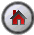SHAZAM Computing Probabilities

## Computing Probabilities for Normal Random Variables

The cumulative distribution function (CDF) for the standard normal random variable can be computed with the `NCDF` function on the `GENR` command. The command format is:

 ` GENR prob=NCDF(var) `

where `var` is a variable that contains numbers and `prob` is a variable that will contain the probabilities.

If the probability for only one number is required then the `GEN1` command should be used. The command format is:

 ` GEN1 prob=NCDF(z) `

where `z` is a number or scalar variable.

The `DISTRIB` command can be used for the calculation of the properties of a variety of probability distributions. For the normal distribution, the general format of the `DISTRIB` command is:

 ` DISTRIB var / TYPE=NORMAL options `

where `var` is a variable with values and `options` is a list of desired options. The option `TYPE=NORMAL` specifies that the normal distribution is required. Some useful options are:

` INVERSE ` Computes critical values of the distribution. The data in the `var` variable must be entered in probabilities.
` MEAN= ` Specifies the value of the population mean. For the normal distribution the default is `MEAN=0`.
` VAR= ` Specifies the value of the population variance. For the normal distribution the default is `VAR=1`.

##### Options that save results
` CDF= ` Saves the values of the cumulative distribution function in the variable specified.
` CRITICAL= ` Saves the critical values in the variable specified when the `INVERSE` option is used.

#### Example

This example is from an exercise in Newbold [1995, Exercise 19, Chapter 5]. Suppose that anticipated consumer demand for a product next month can be represented by a normal random variable with mean 1200 units and standard deviation 100 units.

Denote the random variable by X. For some value x, the CDF is P(X<x)=F(x). The random variable Z=(X`-`1200)/100 has a standard normal distribution (mean 0 and variance 1).

##### Questions and Solutions

(a) What is the probability that sales will exceed 1000 units ? We need to find:

```         P(X > 1000) = 1 - P(X < 1000)
= 1 - F(1000)
```

Note that:

```         P(X < 1000) = P(Z < (1000-1200/100) )
= P(Z < -2)
```

(b) What is the probability that sales will be between 1100 and 1300 units ? We need to find:

```         P(1100 < X < 1300) = P(X < 1300) - P(X < 1100)
= F(1300) - F(1100)
```

Note that:

```         P(X < 1100) = P(Z < (1100-1200/100) ) = P(Z < -1)     and

P(X < 1300) = P(Z < (1300-1200/100) ) = P(Z < 1)
```

(c) The probability is 0.10 that sales will be more than how many units ? We need to find a value b such that:

```         P(X > b) = .10
```

The SHAZAM commands (filename: `NPROB.SHA`) that follow show how to compute the answers. Note that the `GEN1` command is used for scalar arithmetic.

 ```SAMPLE 1 1 * Part (a) GEN1 ANSWER = 1 - NCDF((1000-1200)/100) PRINT ANSWER * Part (b) GEN1 ANSWER = NCDF((1300-1200)/100) - NCDF((1100-1200)/100) PRINT ANSWER * Part (c) * Compute the variance of X GEN1 SIG2=100**2 GEN1 PROB=.1 DISTRIB PROB / TYPE=NORMAL MEAN=1200 VAR=SIG2 INVERSE CRITICAL=ANSWER PRINT ANSWER STOP ```

The SHAZAM output can be viewed. The answers to the questions are:

(a)   ```P(X > 1000) = 0.977 ```

(b)   ``` P(1100 < X < 1300) = 0.683 ```

(c)   ``` P(X > 1328) = .10 ```[SHAZAM Guide home]

### SHAZAM output

``` |_SAMPLE 1 1
|_* Part (a)
|_GEN1 ANSWER = 1 - NCDF((1000-1200)/100)
.9772499

|_* Part (b)
|_GEN1 ANSWER = NCDF((1300-1200)/100) - NCDF((1100-1200)/100)
.6826895

|_* Part (c)
|_* Compute the variance of X
|_GEN1 SIG2=100**2
|_GEN1 PROB=.1
|_DISTRIB PROB / TYPE=NORMAL MEAN=1200 VAR=SIG2 INVERSE CRITICAL=ANSWER
NORMAL DISTRIBUTION - MEAN=   1200.0     VARIANCE=   10000.

PROBABILITY CRITICAL VALUE   PDF
PROB
ROW     1     .10000      1328.2      .17550E-02[SHAZAM Guide home]